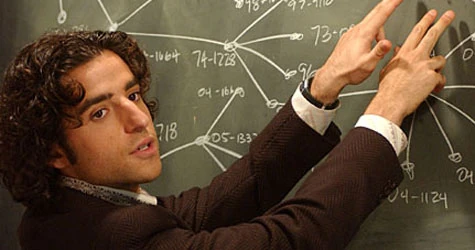# Calorie Counter

You are currently viewing the message boards in:

# Energy required to break covalent bonds of DNA

Posts: 344Member Member
For those of you who fear microwave radiation:

The wavelength of light that is needed to damage DNA lies in the higher energy regions of the electromagnetic spectrum, the higher end of the Ultra-violet (10 x 10^-9 m to 400 x 10^-9 m), the X-Ray (0.01 x 10^-9 to 10 x 10^-9 m), or the Gamma (< 0.02 x 10^-9 m).

Microwave light lies in the low energy region of the electromagnetic spectrum, or 1 mm to 1 m.

Furthermore, the photon energy required to break one of the chemical bonds of DNA, if we use the canonical equations relating the wavelength of light to the energy of a photon:

E = hν

where E is the energy (J, Joules which is a unit of energy), h is Plank's Constant (6.626 × 10-34 J s), and ν is the frequency (s^-1) of the wavelength of light. The frequency is also expressed in terms of the wavelength

ν= c/λ

where c is the speed of light (2.9979 x 10^8 m/s) and λ is the wavelength of light (in meters, m).

So, the energy for a photon is

E = hc/λ

Plugging in the values for the speed of light, Plank's constant, and the higher enery end of the Microwave region, or 1 mm (1 x 10^-3 m), the energy of a photon is

E = [(6.626 x 10^-34 Js)(2.9979 x 10^8 m/s)]/ (1 x 10^-3 m) = 1.986 x 10^-22 J

Similarly, the energy of a photon in the lower and upper regions of the Electromagnetic spectrum are

E = [(6.626 x 10^-34 Js)(2.9979 x 10^8 m/s)]/ (400 x 10^-9 m) = 4.966 x 10^-19 J

E = [(6.626 x 10^-34 Js)(2.9979 x 10^8 m/s)]/ (10 x 10^-9 m) = 1.986 x 10^-17 J.

The energy required to break the covalent bonds (the class of bond that exists between all of the atoms in DNA), the most common being carbon-hydrogen (C-H), carbon-carbon (C-C), and carbon-nitrogen (C-N) have bond energies 413, 348, and 308 kJ/mol, respectively ( a mol means 6.022 x 10^23 constituent particles or atoms per mol; a mol is a unit).

So, the energy in the weakest bond (in this case the carbon-nitrogen bond) is

E = (308 x 10^3 J/mol)/(6.022 x 10^23 mol^-1) = 5.115 x 10^-19 J

THEREFORE, you need to be in the Ultra-violet region of the electromagnetic spectrum.
«1

## Replies

• Posts: 196Member Member• Posts: 782Member Member
Okay. Now the subject makes sense. I was originally pretty stumped by why we were talking about covalent bonds on MFP.
• Posts: 4,061Member Member
Waves are too big
• Posts: 28,493Member Member
I like it...I have no idea what it says, but I like it!
• Posts: 9,175Member Member• Posts: 4,785Member Member
Now you've got me all hot and bothered
• I was just thinking the same thing
• Posts: 2,853Member Member• Posts: 2,599Member Member
I don't fear microwave radiation but I would fear a test on that^.
• Posts: 6,153Member Member
I use microwaves to heat hot toddies.
• Posts: 13,349Member Member
I hope this means that microwaves are safe. Lots of crazy threads on microwaves lately.• Posts: 3,550Member Member
There are these numbers and letters n stuff...and they mean really cool things...and then bad people go to jail, and good people live forever and eat out of microwaves!!!!I don't fear microwaves, but I fear engineers and anyone else who thought differential equations and linear algebra were "fun" courses.
• Posts: 9,175Member Member
I don't fear microwaves, but I fear engineers and anyone else who thought differential equations and linear algebra were "fun" courses.

I'm an engineer. DiffEq was NOT fun. Just for the record.

Physical chemistry was fun. We blew stuff up.
I don't fear microwaves, but I fear engineers and anyone else who thought differential equations and linear algebra were "fun" courses.

I'm an engineer. DiffEq was NOT fun. Just for the record.

Physical chemistry was fun. We blew stuff up.

Oh good! You're infinitely less frightening than the engineers I've known irl. I'm not big on blowing things up-but to each his or her own-as long as we agree that differential equations is not a good time-then we have common ground.
• Posts: 1,927Member Member
I don't fear microwaves, but I fear engineers and anyone else who thought differential equations and linear algebra were "fun" courses.

I'm an engineer. DiffEq was NOT fun. Just for the record.

Physical chemistry was fun. We blew stuff up.

Magnesium?
• Posts: 2,769Member Member
So, you can put the cat in there . . . .
• Ok, I don't know squat about chemistry and physics aside E=MC2... But this thread is getting really interesting!• Posts: 326Member Member
I am invoking the Heisenberg uncertainty principle as well as the second law of thermodynamics in understanding this.....hmmm,,, seems reasonable.
• Posts: 326Member Member
So, you can put the cat in there . . . .

Schrodingers?